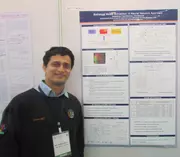X
Statistical compact model extraction: A neural network approach
Pandharpure S.J., Watts J.
Published in IEEE
2012
Volume: 31

Issue: 12
Pages: 1920 - 1924
Abstract
A technique for extracting statistical compact model parameters using artificial neural networks (ANNs) is proposed. ANNs can model a much higher degree of nonlinearity compared to existing quadratic polynomial models and, hence, can even be used in sub-100-nm technologies to model leakage current that exponentially depends on process parameters. Existing techniques cannot be extended to handle such exponential functions. Additionally, ANNs can handle multiple input multiple output relations very effectively. The concept applied to CMOS devices improves the efficiency and accuracy of model extraction. Results from the ANN match the ones obtained from SPICE simulators within 1%. © 1982-2012 IEEE.
•